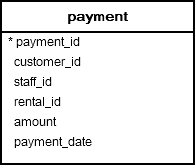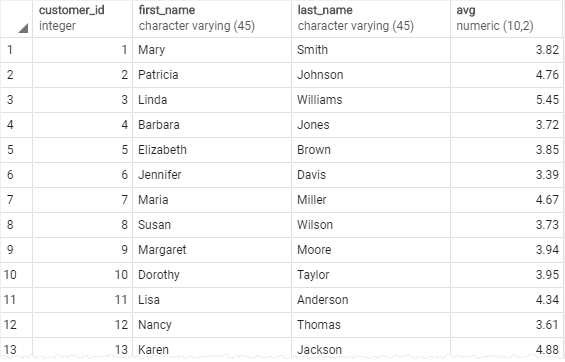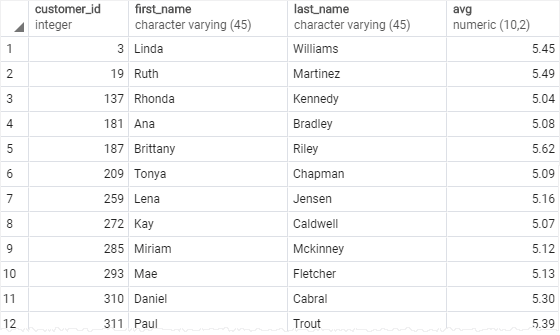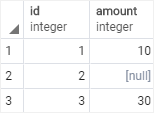# PostgreSQL Tutorial: AVG Function

August 3, 2023

Summary: in this tutorial, you will learn how to use PostgreSQL `AVG()` function to calculate the average value of a set.

## Introduction to PostgreSQL `AVG()` function

The `AVG()` function is one of the most commonly used aggregate functions in PostgreSQL. The `AVG()` function allows you to calculate the average value of a set.

The syntax of the `AVG()` function is as follows:

``````AVG(column)
``````

You can use the `AVG()` function in the `SELECT` and `HAVING`clauses.

Let’s take a look at some examples of using the `AVG` function.

We will use the following `payment` table in the dvdrental sample database for demonstration:## PostgreSQL `AVG()` function examples

If you want to know the average amount that customers paid, you can apply the `AVG` function on the `amount` column as the following query:

``````SELECT AVG(amount)
FROM payment;
``````
``````        avg
--------------------
4.2006056453822965
(1 row)
``````

To make the output more readable, you can use the cast operator as follows:

``````SELECT AVG(amount)::numeric(10,2)
FROM payment;
``````
`````` avg
------
4.20
(1 row)
``````

### PostgreSQL `AVG()` function with `DISTINCT` operator

To calculate the average value of distinct values in a set, you use the distinct option as follows:

``````AVG(DISTINCT column)
``````

The following query returns the average payment made by customers. Because we use `DISTINCT`, PostgreSQL only takes unique amounts and calculates the average.

``````SELECT AVG(DISTINCT amount)::numeric(10,2)
FROM payment;
``````
`````` avg
------
6.14
(1 row)
``````

Notice that the result is different from the first example that does not use the `DISTINCT` option.

### PostgreSQL AVG function with SUM function

The following query uses both `SUM` and `AVG` functions to calculate the total payment made by customers and the average of all transactions.

``````SELECT
AVG(amount)::numeric(10,2),
SUM(amount)::numeric(10,2)
FROM
payment;
``````
`````` avg  |   sum
------+----------
4.20 | 61312.04
(1 row)
``````

### PostgreSQL `AVG()` function with `GROUP BY` clause

To calculate the average value of a group, you use the `AVG` function with GROUP BY clause. First, the `GROUP BY` clause divides rows of the table into groups, the `AVG` function is then applied to each group.

The following example uses the `AVG()` function with `GROUP BY` clause to calculate the average amount paid by each customer:

``````SELECT
customer_id,
first_name,
last_name,
AVG (amount)::NUMERIC(10,2)
FROM
payment
INNER JOIN customer USING(customer_id)
GROUP BY
customer_id
ORDER BY
customer_id;
``````In the query, we joined the `payment` table with the `customer` table using inner join. We used `GROUP BY` clause to group customers into groups and applied the `AVG()` function to calculate the average per group.

### PostgreSQL `AVG()` function with `HAVING` clause

You can use the `AVG` function in the `HAVING` clause to filter the group based on a certain condition. For example, for all customers, you can get the customers who paid the average payment bigger than 5 USD. The following query helps you to do so:

``````SELECT
customer_id,
first_name,
last_name,
AVG (amount)::NUMERIC(10,2)
FROM
payment
INNER JOIN customer USING(customer_id)
GROUP BY
customer_id
HAVING
AVG (amount) > 5
ORDER BY
customer_id;
``````This query is similar to the one above with an additional `HAVING` clause. We used `AVG` function in the `HAVING` clause to filter the groups that have an average amount less than or equal to 5.

### PostgreSQL `AVG()` function and NULL

Let’s see the behavior of the `AVG()` function when its input has NULL.

First, create a table named `t1`.

``````CREATE TABLE t1 (
id serial PRIMARY KEY,
amount INTEGER
);
``````

Second, insert some sample data:

``````INSERT INTO t1 (amount)
VALUES
(10),
(NULL),
(30);
``````

The data of the `t1` table is as follows:

``````SELECT	* FROM 	t1;
``````Third, use the `AVG()` function to calculate average values in the amount column.

``````SELECT AVG(amount)::numeric(10,2)
FROM t1;
``````
``````  avg
-------
20.00
(1 row)
``````

It returns 20, meaning that the `AVG()` function ignores `NULL` values.

In this tutorial, you have learned how to use the PostgreSQL `AVG()` function to calculate the average value of a set.